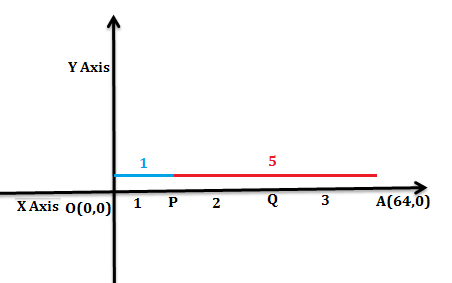QuestionAnswers

# Let O be the origin and A be the point (64, 0). If P and Q divide OA in the ratio $1:2:3$, find the coordinate of the point P.  (a) $\left( \dfrac{32}{3},0 \right)$  (b) $\left( 32,0 \right)$  (c) $\left( \dfrac{64}{3},0 \right)$ (d) $\left( 16,0 \right)$ (e) $\left( \dfrac{16}{3},0 \right)$

Hint: To find the coordinate of the point P by using the section formula. Section formula is used to determine the coordinate of a point that divides a line into two parts such that the ratio of their length is m: n.

Complete step-by-step solution -
Let O be the origin and the coordinate of the point A is (64, 0). The points P and Q divide the line OA in the ratio 1:2:3 as shown in the below figure.Here, O and A be the given two points $\left( {{x}_{1}},{{y}_{1}} \right)=\left( 0,0 \right)$ and $\left( {{x}_{2}},{{y}_{2}} \right)=\left( 64,0 \right)$ respectively and P be the point dividing the line-segment OA internally in the ratio m:n = 1:5, then from the sectional formula for determining the coordinate for a point P is given as
$P(x,y)=\left( \dfrac{m{{x}_{2}}+n{{x}_{1}}}{m+n},\dfrac{m{{y}_{2}}+n{{y}_{1}}}{m+n} \right)................(1)$
Now put all the values in the equation (1), we get
$P(x,y)=\left( \dfrac{1\times 64+5\times 0}{1+5},\dfrac{1\times 0+5\times 0}{1+5} \right)$
$P(x,y)=\left( \dfrac{64+0}{6},\dfrac{0+0}{6} \right)$
$P(x,y)=\left( \dfrac{64}{6},0 \right)$
$P(x,y)=\left( \dfrac{32}{3},0 \right)$
Hence the coordinate of the point P is $\left( \dfrac{32}{3},0 \right)$
Therefore the correct option for the given question is option (a).
Note: The possibility for the mistake is that you might get confused about the difference between an internal and an external division of a line segment. When the point P lies on the external part of the line segment, we use the section formula for the external division for its coordinates. On the contrary, when the point P lies on the internal part of the line segment, we use the section formula for the internal division for its coordinates.